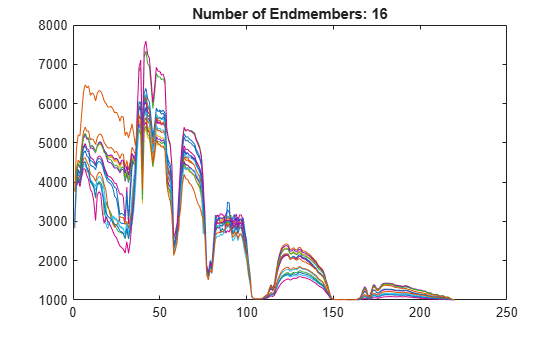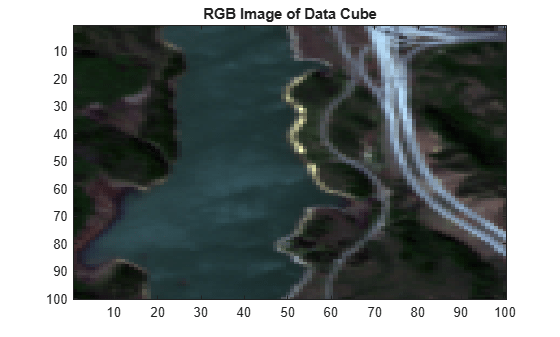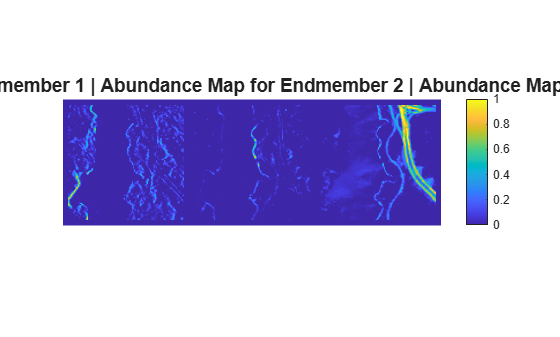# estimateAbundanceLS

Estimate abundance maps

## Syntax

``abundanceMap = estimateAbundanceLS(inputData,endmembers)``
``abundanceMap = estimateAbundanceLS(___,'Method',estMethod)``

## Description

example

````abundanceMap = estimateAbundanceLS(inputData,endmembers)` estimates the abundance maps of the endmembers in a hyperspectral data cube by using the least-squares method.A hyperspectral data cube can contain both pure and mixed pixels. Pure pixels exhibit the spectral characteristics of a single class, while the mixed pixels exhibit the spectral characteristics of multiple classes. The spectral signatures of the pure pixels comprise the endmembers that identify the unique classes present in a hyperspectral data cube. The spectral signature of mixed pixels can be a linear combination of two or more endmember spectra. The abundance map identifies the proportion of each endmember present in the spectra of each pixel. For a hyperspectral data cube of spatial dimensions M-by-N containing P endmembers, there exist P abundance maps, each of size M-by-N.The abundance map estimation process is known as spectral unmixing, which is the decomposition of the spectra of each pixel into a given set of endmember spectra.```

example

````abundanceMap = estimateAbundanceLS(___,'Method',estMethod)` specifies the least-squares method to use for estimating the abundance maps. NoteThis function requires the Image Processing Toolbox™ Hyperspectral Imaging Library. You can install the Image Processing Toolbox Hyperspectral Imaging Library from Add-On Explorer. For more information about installing add-ons, see Get and Manage Add-Ons. ```

## Examples

collapse all

Load a MAT-file containing a hyperspectral data cube and endmember signatures into the workspace. Extract the data cube and endmember signatures.

```data = load('indian_pines.mat'); dataCube = data.indian_pines; endmembers = data.signatures;```

Plot the endmember spectra.

```plot(endmembers) numEndMem = num2str(size(endmembers,2)); title(['Number of Endmembers:' numEndMem]) ```Estimate the abundance maps for the endmember spectra.

`abundanceMap = estimateAbundanceLS(dataCube,endmembers);`

Display the abundance map for each endmember spectra. The order of the abundance maps is the same as the order of the endmembers in the `endmembers` input.

```montage(abundanceMap,'Size',[4 4],'BorderSize',[10 10]); colormap default title('Abundance Maps for Endmembers');```Read hyperspectral data into the workspace.

`hcube = hypercube('jasperRidge2_R198.hdr');`

Extract six endmembers from the hyperspectral data.

`endmembers = nfindr(hcube,6);`

Estimate the abundance map for each endmember using the fully constrained least squares method.

`abundanceMap = estimateAbundanceLS(hcube.DataCube,endmembers,'Method','fcls');`

Estimate an RGB image of the data cube using the `colorize` function.

`rgbImg = colorize(hcube,'Method','RGB');`

Display the RGB image.

```figure imagesc(rgbImg) title('RGB Image of Data Cube')```Display the abundance maps for the endmember spectra. The order of the abundance maps is the same as the order of the endmembers in the `endmembers` input argument.

```figure montage(abundanceMap(:,:,1:3),'Size',[1 3],'BorderSize',[20 20]) colormap default colorbar title('Abundance Map for Endmember 1 | Abundance Map for Endmember 2 | Abundance Map for Endmember 3','FontSize',14)``````figure montage(abundanceMap(:,:,4:6),'Size',[1 3],'BorderSize',[20 20]) colormap default colorbar title('Abundance Map for Endmember 4 | Abundance Map for Endmember 5 | Abundance Map for Endmember 6','FontSize',14)```## Input Arguments

collapse all

Input hyperspectral data, specified as a 3-D numeric array that represent the hyperspectral data cube of size M-by-N-by-C or `hypercube` object. If the input is a `hypercube` object, the function reads the data cube stored in the `DataCube` property of the object. The hyperspectral data cube must be real and non-sparse.

Data Types: `single` | `double` | `int8` | `int16` | `int32` | `int64` | `uint8` | `uint16` | `uint32` | `uint64`

Endmember signatures, specified as a matrix of size C-by-P. where C is the number of spectral bands in the input hyperspectral data and P is the number of endmember spectral signatures.

Data Types: `single` | `double` | `int8` | `int16` | `int32` | `int64` | `uint8` | `uint16` | `uint32` | `uint64`

Method for estimating abundance maps, specified as one of these values.

• `'ucls'` — Unconstrained least-squares method.

• `'fcls'` — Fully constrained least-squares method.

• `'ncls'` — Nonnegative constrained least-squares method.

Example: `estimateAbundanceLS(inputData,endmembers,'Method','ncls')`

Data Types: `char` | `string`

## Output Arguments

collapse all

Abundance maps, returned as a 3-D numeric array of size M-by-N-by-P.

Data Types: `double`

 Keshava, N., and J.F. Mustard. “Spectral Unmixing.” IEEE Signal Processing Magazine 19, no. 1 (January 2002): 44–57. https://doi.org/10.1109/79.974727.

 Kay, Steven M. Fundamentals of Statistical Signal Processing. Prentice Hall Signal Processing Series. Englewood Cliffs, N.J: Prentice-Hall PTR, 1993.